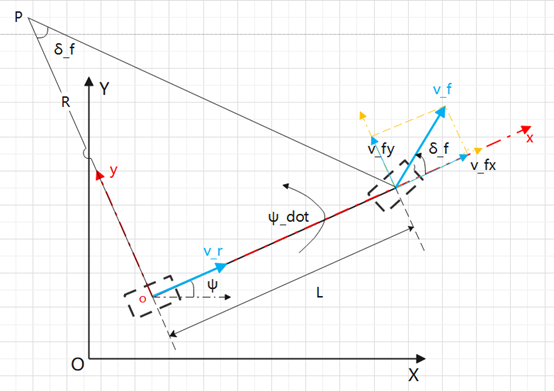## 一、车辆运动学模型概述

《Vehicle Dynamics and Control》书中，将机体坐标系原点设置在质心位置，假设前后轮都存在偏角，推导了一般方程。

《无人驾驶车辆模型预测控制》书中，将机体坐标系原点设置在后轴中心，假设只存在前轮偏角，推导了以后轴为原点的运动学模型。

## 二、以后轴中心为原点的车辆运动学模型推导y轴方向：## 三、matlab实现

function vehicle_state_new = kinematic_state(vehicle_state, x, dt)

% 状态量
X = vehicle_state(1,1);      % 水平位移 m 大地坐标系
Y = vehicle_state(1,2);      % 垂直位移 m 大地坐标系
Psi = vehicle_state(1,3);    % 航向角 rad 大地坐标系
% 输入量
delta = x(1,1);              % steering wheel
v_r = x(1,2);                % pedal
% 车辆参数
len_wheel = 2.7;             % 车辆轴距 m

% 状态更新
Psi_new = Psi + v_r * tan(delta) / len_wheel;
X_new = X + v_r * cos(Psi_new) * dt;
Y_new = Y + v_r * sin(Psi_new) * dt;

vehicle_state_new = [X_new, Y_new, Psi_new];

end